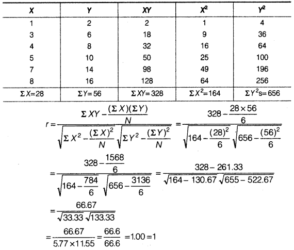# Chapter 7 – Correlation Questions and Answers: NCERT Solutions for Class 11 Economics(Statistics for Economics)

Class 11 Economics(Statistics for Economics) NCERT book solutions for Chapter 7 - Correlation Questions and Answers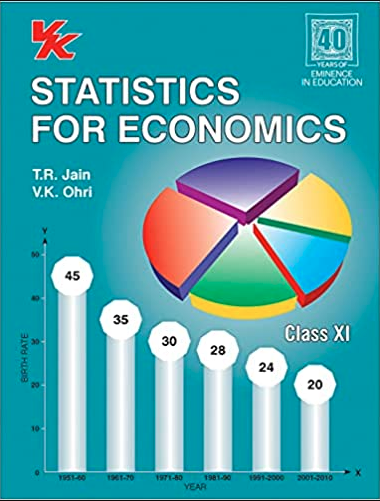## Question 16. Calculate the correlation coefficient between the heights of fathers in inches (X) and their sons (Y).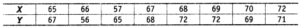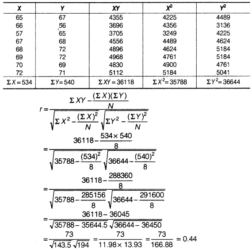## Question 17. Calculate the correlation coefficient between X and Y and comment on their relationship.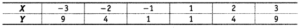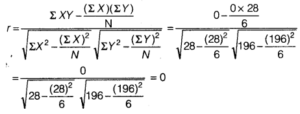## Question 18. Calculate the correlation coefficient between X and Y and comment on their relationship.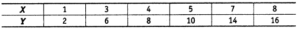### Solution